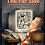## Tuesday, March 8, 2011

### Difference between \$* and \$@ in Shell

In one of our earlier articles, we saw the different positional parameters in a shell script. We discussed about the positional parameter \$*. There is one more positional parameter, \$@, the definition of which is also the same as \$*. Let us see in this article the exact difference between the parameters \$* and \$@.

First, let us write a simple shell script to understand \$@:
```\$ cat cmd
#!/usr/bin/bash

echo "The total no of args are: \$#"
echo "The \\$* is: \$*"
echo "The \\$@ is: \$@"
```
On running the above program with some command line arguments:
```\$ ./cmd 1 2 3
The total no of args are: 3
The \$* is: 1 2 3
The \$@ is: 1 2 3
```
As shown in the above output, both the \$* and \$@ behave the same. Both contain the command line arguments given.

Let us now write a script which exactly shows the difference:
```\$ cat cmd
#!/usr/bin/bash

echo "Printing \\$* "
for i in \$*
do
echo i is: \$i
done

echo "Printing \\$@ "
for i in "\$@"
do
echo i is: \$i
done
```
Now, on running the above script:
```\$ ./cmd a b "c d" e
Printing \$*
i is: a
i is: b
i is: c
i is: d
i is: e
Printing \$@
i is: a
i is: b
i is: c d
i is: e
```
In the above example, we write a for loop and display the arguments one by one using \$* and \$@. Notice the difference. When we pass the command line argument in double quotes("c d"), the \$* does not consider them as a single entity, and splits them. However, the \$@ considers them as a single entity and hence the 3rd echo statement shows "c d" together. This is the difference between \$* and \$@.

1.I'm just a n00b, but here goes.
I got 4 different result, depending if I quoted \$* or \$@ in the for loop:

script cmd2 running:

>cmd2 1 2 3 4 5 "6 7 8" 9
Printing \$*
i is: 1
i is: 2
i is: 3
i is: 4
i is: 5
i is: 6
i is: 7
i is: 8
i is: 9
Printing \$@
i is: 1
i is: 2
i is: 3
i is: 4
i is: 5
i is: 6
i is: 7
i is: 8
i is: 9
Printing "\$*"
i is: 1 2 3 4 5 6 7 8 9
Printing "\$@"
i is 1
i is 2
i is 3
i is 4
i is 5
i is 6 7 8
i is 9

script cmd2 listing:

#! /bin/bash

## No quotes \$*
echo "Printing \\$* "
for i in \$*
do
echo i is: \$i
done

## No quotes \$@
echo "Printing \\$@ "
for i in \$@
do
echo i is: \$i
done

## Quoted "\$*"
echo "Printing \"\\$*\" "
for i in "\$*"
do
echo i is: \$i
done

## Quoted "\$@"
echo "Printing \"\\$@\" "
for i in "\$@"
do
echo i is \$i
done

exit

2.\$* if quoted will always give the entire command line argument list as a single argument.

\$@, if not quoted, will behave like \$*.